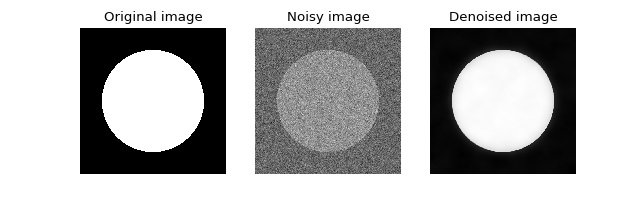# Image denoising (Douglas-Rachford, Total Variation, L2-norm)¶

This tutorial presents an image denoising problem solved by the Douglas-Rachford splitting algorithm. The convex optimization problem, a term which expresses a prior on the smoothness of the solution constrained by some data fidelity, is given by

$\min\limits_x \|x\|_\text{TV} \text{ s.t. } \|x-y\|_2 \leq \epsilon$

where $$\|\cdot\|_\text{TV}$$ denotes the total variation, y are the measurements and $$\epsilon$$ expresses the noise level.

Create a white circle on a black background

>>> import numpy as np
>>> N = 650
>>> im_original = np.resize(np.linspace(-1, 1, N), (N, N))
>>> im_original = np.sqrt(im_original**2 + im_original.T**2)
>>> im_original = im_original < 0.7


and add some random Gaussian noise

>>> sigma = 0.5  # Variance of 0.25.
>>> np.random.seed(7)  # Reproducible results.
>>> im_noisy = im_original + sigma * np.random.normal(size=im_original.shape)


The prior objective function to minimize is defined by

$f_1(x) = \|x\|_\text{TV}$

which can be expressed by the toolbox TV-norm function object, instantiated with

>>> from pyunlocbox import functions
>>> f1 = functions.norm_tv(maxit=50, dim=2)


The fidelity constraint expressed as an objective function to minimize is defined by

$f_2(x) = \iota_S(x)$

where $$\iota_S()$$ is the indicator function of the set $$S = \left\{z \in \mathbb{R}^n \mid \|z-y\|_2 \leq \epsilon \right\}$$ which is zero if $$z$$ is in the set and infinite otherwise. This function can be expressed by the toolbox L2-ball function, instantiated with

>>> y = np.reshape(im_noisy, -1)  # Reshape the 2D image as a 1D vector.
>>> epsilon = N * sigma           # Variance multiplied by N^2.
>>> f = functions.proj_b2(y=y, epsilon=epsilon)
>>> f2 = functions.func()
>>> f2._eval = lambda x: 0        # Indicator functions evaluate to zero.
>>> def prox(x, step):
...     return np.reshape(f.prox(np.reshape(x, -1), 0), im_noisy.shape)
>>> f2._prox = prox


Note

We defined a custom proximal operator which transforms the 2D image as a 1D vector because pyunlocbox.functions.proj_b2 operates on the columns of x while pyunlocbox.functions.norm_tv needs a two-dimensional array to compute the 2D TV norm.

The Douglas-Rachford splitting algorithm is instantiated with

>>> from pyunlocbox import solvers
>>> solver = solvers.douglas_rachford(step=0.1)


and the problem solved with

>>> x0 = np.array(im_noisy)  # Make a copy to preserve y aka im_noisy.
>>> ret = solvers.solve([f1, f2], x0, solver)
Solution found after 25 iterations:
objective function f(sol) = 2.080376e+03
stopping criterion: RTOL


Let’s display the results:

>>> import matplotlib.pyplot as plt
>>> fig = plt.figure(figsize=(8, 2.5))
>>> ax1 = fig.add_subplot(1, 3, 1)
>>> _ = ax1.imshow(im_original, cmap='gray')
>>> _ = ax1.axis('off')
>>> _ = ax1.set_title('Original image')
>>> ax2 = fig.add_subplot(1, 3, 2)
>>> _ = ax2.imshow(im_noisy, cmap='gray')
>>> _ = ax2.axis('off')
>>> _ = ax2.set_title('Noisy image')
>>> ax3 = fig.add_subplot(1, 3, 3)
>>> _ = ax3.imshow(ret['sol'], cmap='gray')
>>> _ = ax3.axis('off')
>>> _ = ax3.set_title('Denoised image')The above figure shows a good reconstruction which is both smooth (the TV prior) and close to the measurements (the L2 fidelity constraint).